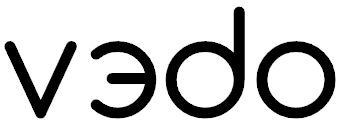Fork me on GitHub

A python module for scientific analysis and visualization of эd objects

## Features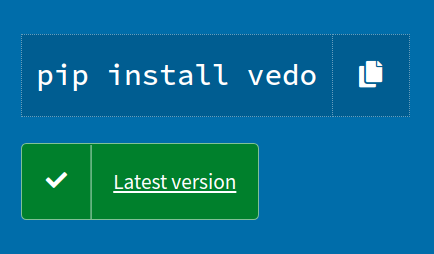## Quick Start

To visualize a file from a web URL (or from your Dropbox!), simply type in your terminal:

``````pip install vedo
vedo https://vedo.embl.es/examples/data/panther.stl.gz``````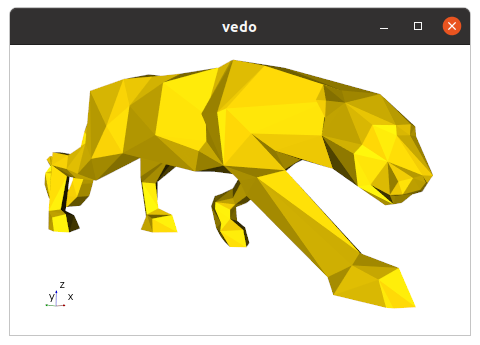To visualize a full 3D interactive scene, type:

``vedo https://vedo.embl.es/examples/geo_scene.npz``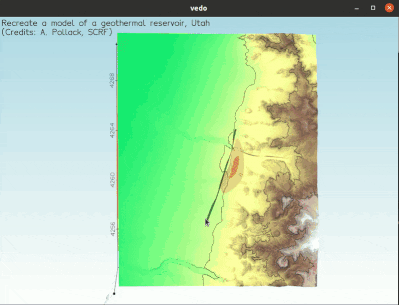Let's create an interactive 3D scene with a simple cone, in 3 lines of python code:

``````from vedo import Cone

# Create a simple cone
c = Cone()

# Show it (with axes)
c.show(axes=1)

# Now you can interact with the 3D scene,
# Press "h" and explore the possibilities! ``````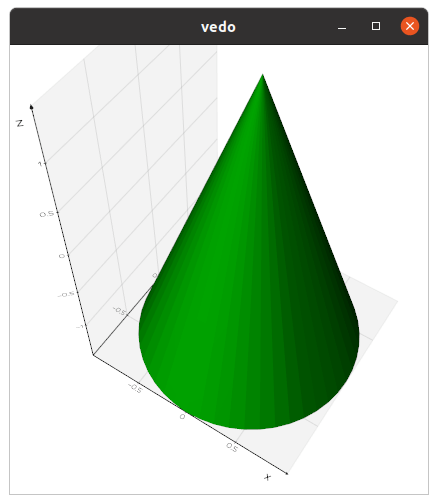Let's write a simple python script that loads a polygonal Mesh and generates a cool rendering by adding some custom light sources to the scene:

``````from vedo import *

# Load a polygonal mesh, make it white and glossy:
man = Mesh('https://vedo.embl.es/example/data/man.vtk')
man.c('white').lighting('glossy')

# Create two points:
p1 = Point([ 1,0,1], c='yellow')
p2 = Point([-1,0,2], c='red')

# Add colored light sources at the point positions:
l1 = Light(p1, c='yellow')
l2 = Light(p2, c='red')

# Show everything in one go:
show(man, l1, l2, p1, p2, "Hello World", axes=True)``````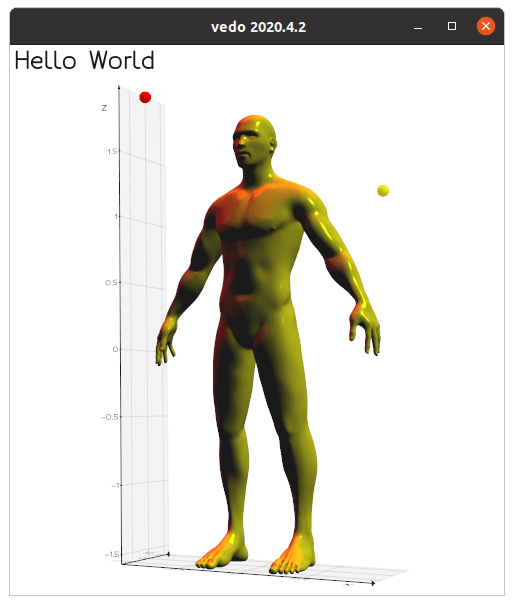Let's create a Volume - a volumetric dataset - from a numpy object:

``````import numpy as np
from vedo import *

# Create a scalar field: the distance from point (15,15,15)
X, Y, Z = np.mgrid[:30, :30, :30]
scalar_field = ((X-15)**2 + (Y-15)**2 + (Z-15)**2)/225

# Create the Volume from the numpy object
vol = Volume(scalar_field)

# Generate the surface that contains all voxels in range [1,2]

show(lego, axes=True)``````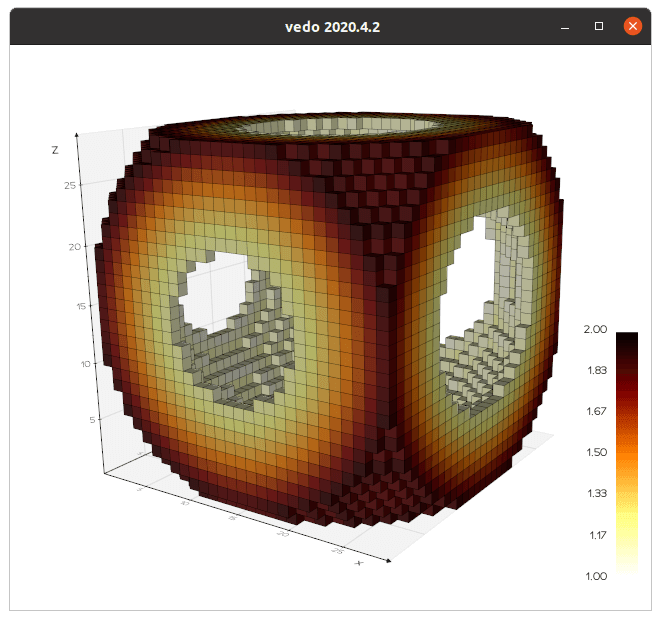Draw 3D scatter plot plot of oriented wind speed directions:

``````import numpy as np
import pandas as pd
from vedo import Cone, Glyph, show

pts = np.c_[df['x'], df['y'], df['z']]
vecs= np.c_[df['u'], df['v'], df['w']]

# Create a mesh to be used like a symbol (a "glyph") to be attached to each point
cone = Cone().scale(0.3).rotateY(90)  # make it smaller and orient tip to positive x

glyph = Glyph(pts, cone, vecs, scaleByVectorSize=True, colorByVectorSize=True)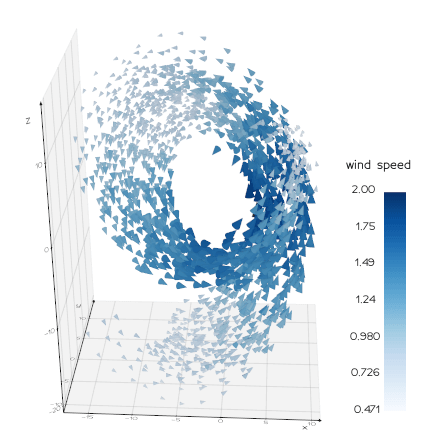### References

Scientific publications leveraging `vedo`:
• X. Diego et al.: "Key features of Turing systems are determined purely by network topology", Physical Review X, 20 June 2018.
• M. Musy, K. Flaherty et al.: "A Quantitative Method for Staging Mouse Limb Embryos based on Limb Morphometry", Development, 5 April 2018, DOI.
• F. Claudi, A. L. Tyson, T. Branco, "Brainrender. A python based software for visualisation of neuroanatomical and morphological data.", DOI.
• J. S. Bennett, D. Sijacki, "Resolving shocks and filaments in galaxy formation simulations: effects on gas properties and star formation in the circumgalactic medium", Monthly Notices of the Royal Astronomical Society, Volume 499, Issue 1, November 2020, DOI.
• G. Dalmasso et al., "Evolution in space and time of 3D volumetric images", in preparation.
• A. Pollack et al., "Stochastic inversion of gravity, magnetic, tracer, lithology, and fault data for geologically realistic structural models: Patua Geothermal Field case study" Geothermics Volume 95, September 2021, DOI.
• X. Lu et al., "3D electromagnetic modeling of graphitic faults in the Athabasca Basin using a finite-volume time-domain approach with unstructured grids", June 2021, DOI.
Presentations at international conferences:
• M. Musy, G. Dalmasso, J. Sharpe and N. Sime, "`vedo`: plotting in FEniCS with python", Poster at FEniCS'2019, SDTM, Washington DC, June 2019.
• G. Dalmasso, "Evolution in space and time of 3D volumetric images". Talk at Image-based Modeling and Simulation of Morphogenesis. Max Planck Institute for the Physics of Complex Systems, Dresden, Germany, March 2019.
• G. Dalmasso, "A four-dimensional growing mouse limb bud reconstruction". Talk at SEBD, Spain, November 2020.
You can cite `vedo` as:
• M. Musy et al., "`vedo`, a python module for scientific analysis and visualization of 3D objects and point clouds", Zenodo, 2021, doi.org/10.5281/zenodo.4287635.